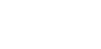# Verification of Clerk Maxwell’s Reciprocal TheoremReading time: 1 minute

To verify clerk- Maxwell's Reciprocal Theorem and to determine the Young's modulus of beam arterial of the apparatus. EQUIPMENT REQUIRED
1. Clerk-Maxwell's reciprocal' theorem apparatus
2. Tire weight
3. Dial gauge
THE THEOREM Clerk-Maxwell's reciprocal theorem state that in a linearly elastic structure, the deflection at any point. A due to a load applied at some other point 13 will be equal to the deflection at B when the same load is applied at A. THE APPARATUS Clerk-Maxwell's reciprocal theorem apparatus consists of a rigid frame and a light beam. The beam is provided with simple end supports over the rigid frame in the form of a hinge at one end and a roller at other end. There is a tire rod assembly to load the beam using tire- weights (Weight of this assembly is made equal to that of one tire —weight). There is also a traveling pedestal to support the dial gauge for measuring deflections. PROCEDURE Place the beam correctly over the supports. Mount the dial gauge on the pedestal, place it under the beam exactly at mid- span and adjust it to read zero on the scale. Hung the tire rod assembles exactly at quarter-span and notes the dial gauge reading. Place the tire weights one by one, noting the dial gauge reading every time a weight is placed. Continue the observations while unloading the beam also. Repeat the process after interchanging the positions of the dial gauge and the tire rod assemble. OBSERVATIONS Record the observations in the tabular form. GRAPH Plot a graph with deflection on X- axis and load on Y- axis for both the case. CALCULATIONS The deflection at quarter point due to at the center is given byWhere  is the deflection, W- the load, L- the span, I — the moment or inertia of the section the beam and E- the Young's of the beam. Hence E can be found out. VERIFICATION 1. Compare the deflection under different loads in case (1) with those in case (2). They will be found to be the same, thus verifying the theorem. 2. Superpose the plot of load Vs deflection or case (I) with that of case (2). They will coincide, thereby, verifying the theorem again. RESULT Verification of Clerk-Maxwell's reciprocal theorem Young's Modulus of the material of the beam= QUESTION – DO YOU KNOW? 1. The law of reciprocal Theorem. 2. What is the type of supports provided in the Clerk-Maxwell's apparatus? 3. Why the depth of beam is less than the width in Maxwell's reciprocal apparatus?